# Complex periodic calculations

The complex periodic calculations mechanism is one of the platform’s applied mechanisms. It allows for the implementation of various models for calculating payroll and is based on two components:

(1) Complex periodic calculations mechanism contains tools for describing different types of calculations that will be used in the applied solution, including salary, alimony, fines, etc. In addition to the actual description of these types of calculation, there is a feature for specifying the rules by which some types of calculations will affect others.

(2) This mechanism provides for the storage of intermediate data used in calculations and the final results of calculations.

The complex periodic calculations mechanism is facilitated by two objects of the applied solution: the Chart of calculation types and the Calculation register.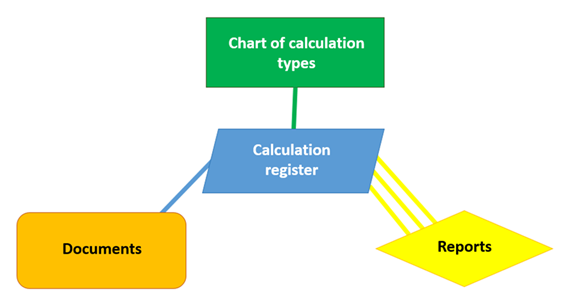• Chart of calculation types
It is used to describe the calculation types and their mutual influence on each other. In an application, an arbitrary number of charts of calculation types, depending on the accounting model, may be implemented spontaneously.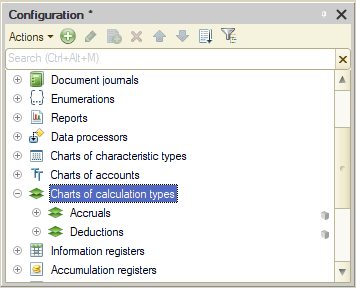• Calculation Register
It is designed for storing records on various types of calculations to be performed, as well as for storing intermediate data and the results of performed calculations. An applied solution may contain several calculation registers for displaying the data of a certain accounting section.

## Chart of calculation types

The charts of calculation types are applied configuration objects that are used in the complex periodic calculations mechanism, which serve to describe the types of calculations and their mutual influence on each other.

#### Structure

A chart of calculation types is a list of calculation types, each with its own code, name and a set of attributes that contain additional self-identifying information: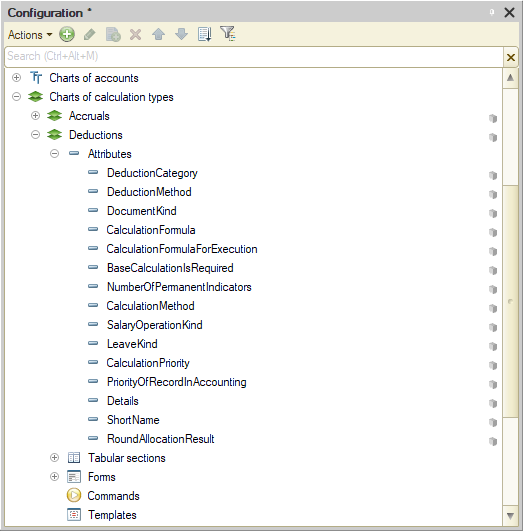For example, an Accruals chart of calculation types might appear as in the screenshot below: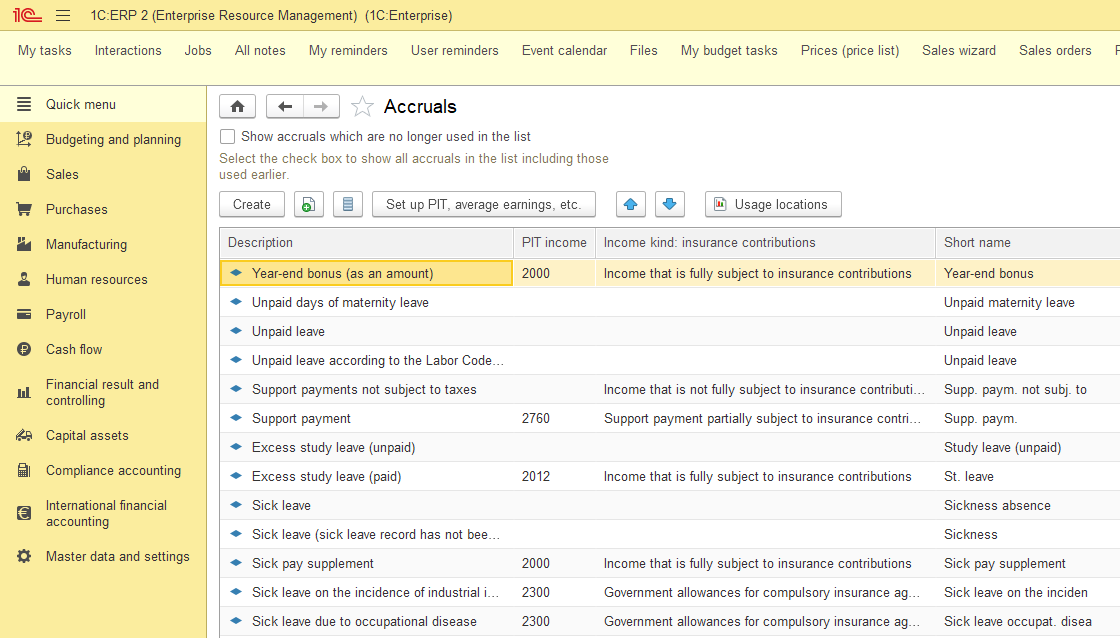The creation and editing of calculation types can be performed by both the developer (predefined calculation types), and the user, during the work process with the applied solution. However, the user cannot delete predefined calculation types created by the developer.

Calculation types created in the chart of calculation types may affect each other. The system supports two influence modes: dependence by the base period and substitution by the validity period.

For each calculation type, you can specify a list of calculation types on which it will depend on the base period, and which will displace it by the validity period.

In addition to these dependencies for a calculation type, the so-called leading calculation types can be indicated, i.e. those on which it does not depend directly, but which can influence it through other types of calculations.

#### Forms of the charts of calculation types

In order to allow the user to view and change the data stored in the chart of calculation types, the system supports multiple forms for its presentation. The system can automatically generate all the necessary forms; yet at the same time, the developers can create their own forms, used instead of the default forms by the system.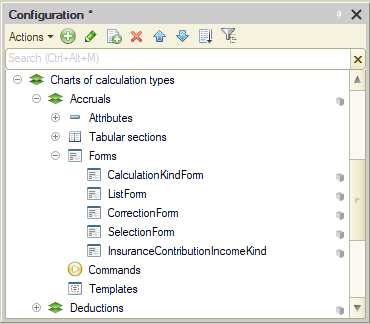#### List form

The calculation types are viewed using the list form, which allows a user to navigate through the list, add, mark and delete calculation types. Also, the list form makes it possible to sort and select the displayed information according to several criteria: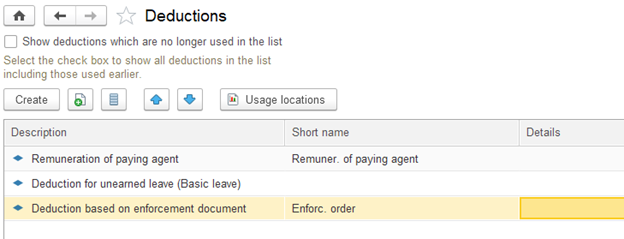#### Calculation type form

The calculation type form is used to view and modify the data of certain calculation types. It usually presents the data in a user-friendly format for reading and editing: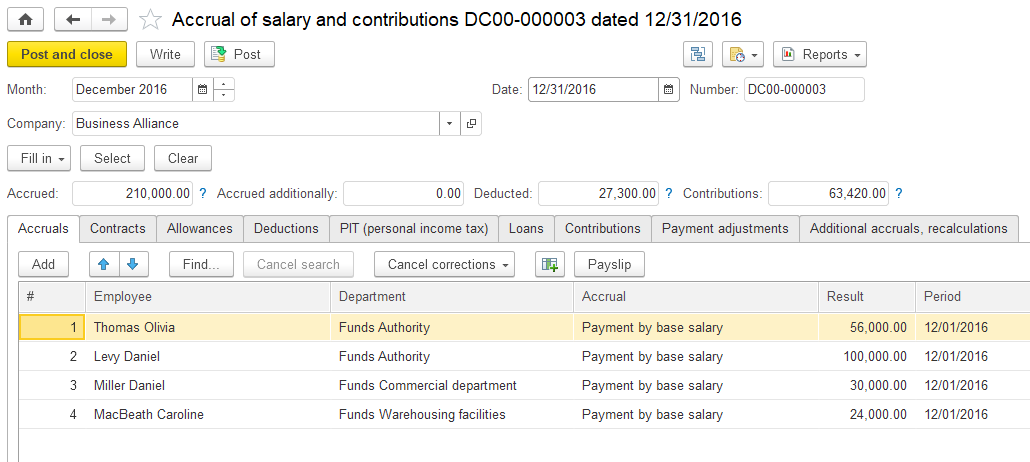In addition to these two forms, the system also supports the form for selecting certain calculation types from the list, which usually contains a minimum set of information needed to select a particular type of calculation.

## Calculation register

The calculation registers are applied configuration objects, used in the complex periodic calculations mechanism for storing records of the various calculations to be performed, as well as for storing intermediate data and the results of the performed calculations.

#### Structure

A calculation register stores the information in the form of records, each of which contains dimension values and corresponding resource values.

The register dimension describes the sections in which the information is stored, and register resources directly contain this information. For example, the Accruals calculation register has the following structure: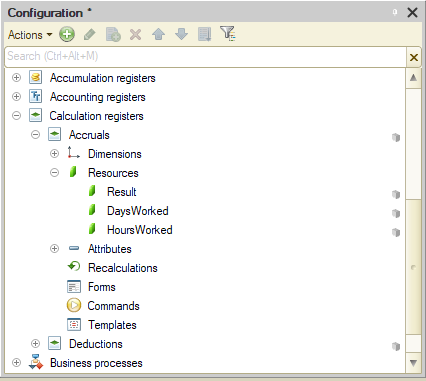Its database records will look like this: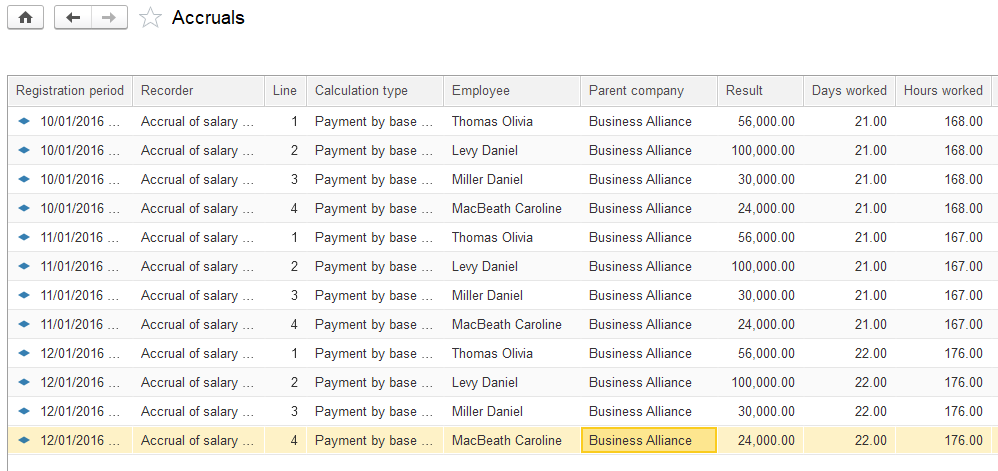#### Relationship to the chart of calculation types

A calculation register is linked to one of the charts of calculation types existing in the applied solution. This relationship necessitates that each register record has a Calculation type field, through which register mechanisms can monitor the mutual influence of the calculation records on each other.

#### Periodicity

A calculation register stores data by both dimension and time. This is the reason why each register record has one more mandatory field – Validity period. When creating a calculation register, the developer can specify the minimum periodicity for entering records in the register: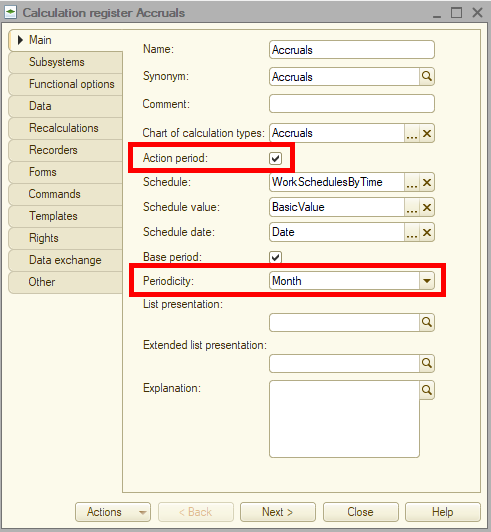#### Submission to the recorder

Document posting, as a rule, causes changes in the calculation register state. Therefore, each register entry record is linked to a particular document, the recorder, and the line number of that document. Adding, modifying or deleting records in the recorder will simultaneously affect all records related to a singular document.

#### Connection with the time graph

We can specify the connection with a time graph for the calculation register. The time graph is an information register that contains a time scheme of the source data involved in calculations. This graph can have such dimensions as, for example, work schedule or date, and such resources as the number of working hours on this date. So, by means of the built-in language, we can connect a calculation register record with a determined work schedule and receive further information on the number of working hours required for performing calculations.

For example, a time graph that has the following structure: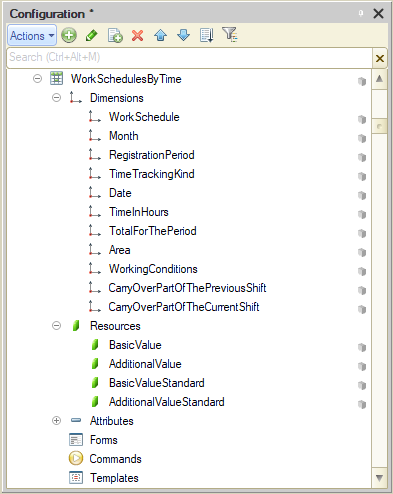may contain information on the number of working hours: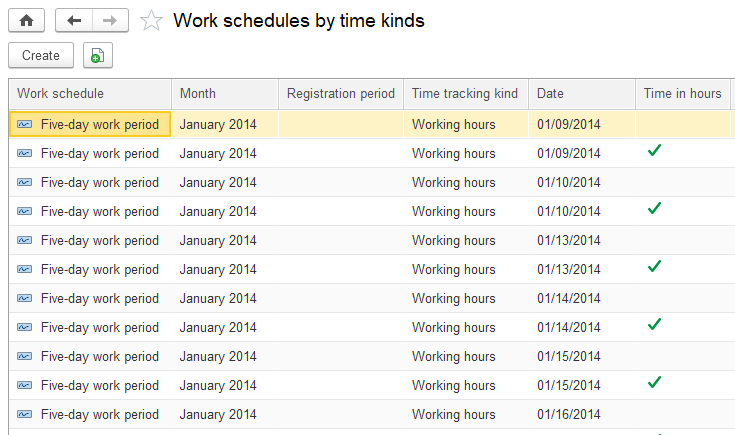#### Recalculations

A calculation register may include special objects – Recalculations.

In these objects, the system stores information on the obsolescent calculation register records that are subject to recalculation as a result of the work of both mechanisms – dependence by the base period and substitution by the validity period.

Uniqueness of Records

The system ensures control over the uniqueness of records stored in the calculation register. Therefore, the calculation register cannot contain two records relating to the same line of the same document.

## Mechanisms implemented by the calculation register

#### Substitution by the validity period

The mechanism of substitution by the validity period facilitates the calculation of the actual validity period of a calculation register record based on the analysis of other records contained in the register.

Generally, a calculation register record contains two dates that determine the period covered by the record validity, which is called the record validity period. However, if the calculation type, to which this record is related, can be substituted by another calculation type, then the validity period of this record is only the “requested” period or “which we want to be valid within this period”. In practice, the actual validity period of this record can be determined only after the analysis of all records of calculation types that substitute this calculation type by the validity period. The actual validity period will be a set of periods that are a subset of the initial validity period of this record. If no records are found that substitute the given one by the validity period, then the actual validity period of this record will be equal to its validity period.

Another extreme case of substitution by the validity period is the complete displacement of a given record by other records. In this case, the actual record validity period will be absent.

Each calculation register record contains a calculation type to which it relates. In order to determine which records should displace the given record by the validity period, the calculation register uses the connection with the chart of calculation types, which describes the intermutual influence of the calculation types on each other. Using this relationship enables the calculation register to determine the actual validity period of each record.

#### Dependence by the base period

The dependence mechanism for the base period allows you to get the base value for the calculation register record based on the analysis of other records stored in the register.

The base is a numerical value that should be used to calculate the result of this record. The base is calculated by analyzing the results of the calculation of other records, on which this record depends according to the base period. Thus, in the general case, the calculation register record contains two dates that determine the period in which it is necessary to analyze the records of calculation types on which this type of calculation depends on the base — the base period. Thus, in general, the calculation register record contains two dates that determine the period within which it is necessary to analyze the records of calculation types on which this calculation type depends by the base — the base period. Using the connection with the chart of calculation types allows the calculation register to determine the calculation types on which this calculation type depends by the base period.

The calculation register supports two types of dependence by the base period:

• dependence by the validity period;

• dependence by the registration period.

In the case of dependency by the validity period, those records for which intersections of their actual validity period with the base period of the given record are found will be selected to obtain the base. The value of the base that will be obtained from the particular influencing record is generally not equal to the result that this record contains. The base will be calculated in proportion to how much of the actual period of the affecting record is the overlapping section with the specified base period. This will use the data from the graph associated with this record.

In case of dependence by the registration period, to obtain the base, the system will select the results of calculating those records that fall into the base period of this record by the value of their field Registration period.

The most difficult variant of the dependence by the base period is the case when the Validity period is the base period property is set for the calculation type of this record. This property means that the base period specified in the corresponding fields of the record will not be used as the base period, but the actual validity period, obtained as a result of the work of the mechanism of substitution by the validity period and which, in general, is a set of some periods.

Recalculation record generation

The mechanism of recalculation record generation tracks the input of new records to the register that affect the result of calculating existing records. The possibility of the influence of new records on existing ones is determined as a result of analysis of the mutual influence of calculation types and on the basis of the operation of the mechanisms of substitution by the validity period and dependence by the base period.

The result of the mechanism for recalculation record generation is a set of recalculation records containing information about register records that should be recalculated.

## Calculation register features

The developer is provided with the following fundamental calculation register features:

• selecting records within the given interval by the specified criteria;

• selecting records by recorders;

• receiving a base value for the register records that satisfy the specified selection;

• receiving graph data for the register records that satisfy the specified selection;

• receiving data on the records that are subject to recalculation;

• viewing, modifying and inputting the set of records to the register.

Be the first to know tips & trick on business application development!